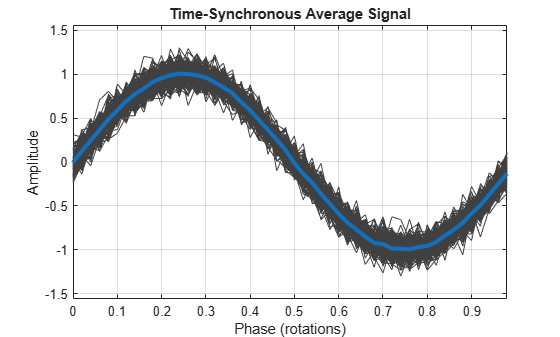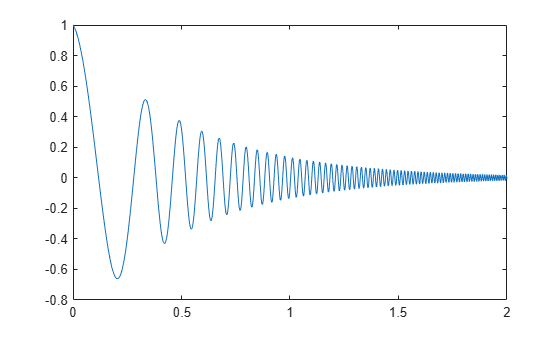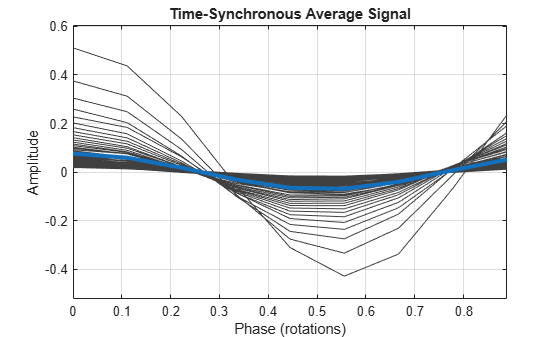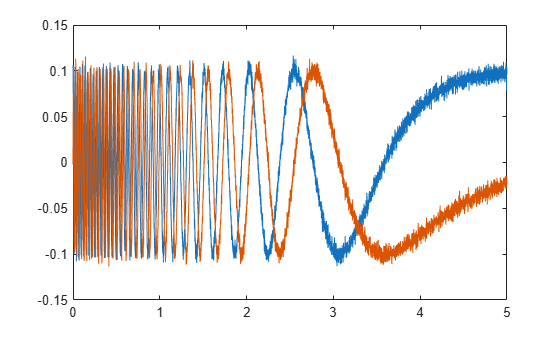Documentation

### This is machine translation

Mouseover text to see original. Click the button below to return to the English version of the page.

Note: This page has been translated by MathWorks. Click here to see
To view all translated materials including this page, select Country from the country navigator on the bottom of this page.

# tsa

Time-synchronous signal average

## Syntax

``ta = tsa(x,fs,tp)``
``ta = tsa(x,t,tp)``
``ta = tsa(xt,tp)``
``ta = tsa(___,Name,Value)``
``[ta,t,p,rpm] = tsa(___)``
``tsa(___)``

## Description

example

````ta = tsa(x,fs,tp)` returns a time-synchronous average of a signal `x`, sampled at a rate `fs`, on the pulse times specified in `tp`.```
````ta = tsa(x,t,tp)` returns a time-synchronous average of `x` sampled at the time values stored in `t`.```

example

````ta = tsa(xt,tp)` returns a time-synchronous average of a signal stored in the MATLAB® timetable `xt`.```

example

````ta = tsa(___,Name,Value)` specifies additional options for any of the previous syntaxes using name-value pair arguments. For example, you can specify the number of tachometer pulses per shaft rotation or choose to average the signal in the time domain or the frequency domain.```
````[ta,t,p,rpm] = tsa(___)` also returns `t`, a vector of sample times corresponding to `ta`; a vector `p` of phase values; and `rpm`, the constant rotational speed (in revolutions per minute) corresponding to `ta`.```
````tsa(___)` with no output arguments plots the time-synchronous average signal and the time-domain signals corresponding to each signal segment in the current figure.```

## Examples

collapse all

Compute the time-synchronous average of a noisy sinusoid.

Generate a signal consisting of a sinusoid embedded in white Gaussian noise. The signal is sampled at 500 Hz for 20 seconds. Specify a sinusoid frequency of 10 Hz and a noise variance of 0.01. Plot one period of the signal.

```fs = 500; t = 0:1/fs:20-1/fs; f0 = 10; y = sin(2*pi*f0*t) + randn(size(t))/10; plot(t,y) xlim([0 1/f0])```Compute the time-synchronous average of the signal. For the synchronizing signal, use a set of pulses with the same period as the sinusoid. Use `tsa` without output arguments to display the result.

```tPulse = 0:1/f0:max(t); tsa(y,fs,tPulse)```Generate a signal that consists of an exponentially damped quadratic chirp. The signal is sampled at 1 kHz for 2 seconds. The chirp has an initial frequency of 2 Hz that increases to 28 Hz after the first second. The damping has a characteristic time of 1/2 second. Plot the signal.

```fs = 1e3; t = 0:1/fs:2; x = exp(-2*t').*chirp(t',2,1,28,'quadratic'); plot(t,x)```Create a duration array using the time vector. Construct a timetable with the duration array and the signal. Determine the pulse times using the locations of the signal peaks. Display the time-synchronous average.

```ts = seconds(t)'; tx = timetable(ts,x); [~,lc] = findpeaks(x,t); tsa(tx,lc)```Compute the time-synchronous average. View the types of the output arguments. The sample times are stored in a duration array.

```[xta,xt,xp,xrpm] = tsa(tx,lc); whos x*```
``` Name Size Bytes Class Attributes x 2001x1 16008 double xp 9x1 1192 timetable xrpm 1x1 8 double xt 9x1 74 duration xta 9x1 1190 timetable ```

Convert the duration array to a `datetime` vector. Construct a timetable using the `datetime` vector and the signal. Compute the time-synchronous average, but now average over sets of 15 rotations.

View the types of the output arguments. The sample times are again stored in a duration array, even though the input timetable used a `datetime` vector.

```dtb = datetime(datevec(ts)); dtt = timetable(dtb,x); nr = 15; tsa(dtt,lc,'NumRotations',nr)``````[dta,dt,dp,drpm] = tsa(dtt,lc,'NumRotations',nr); whos d*```
``` Name Size Bytes Class Attributes dp 135x1 3208 timetable drpm 1x1 8 double dt 135x1 1082 duration dta 135x1 3206 timetable dtb 2001x1 32017 datetime dtt 2001x1 49059 timetable ```

Compute the time-synchronous average of the position of a fan blade as it slows down after switchoff.

A desk fan spinning at 2400 rpm is turned off. Air resistance (with a negligible contribution from bearing friction) causes the fan rotor to stop in approximately 5 seconds. A high-speed camera measures the x-coordinate of one of the fan blades at a rate of 1 kHz.

```fs = 1000; t = 0:1/fs:5-1/fs; rpm0 = 2400;```

Idealize the fan blade as a point mass circling the rotor center at a radius of 10 cm. The blade experiences a drag force proportional to speed, resulting in the following expression for the phase angle:

`$\varphi =2\pi {\mathit{f}}_{0}\mathit{T}\left(1-{\mathit{e}}^{-\mathit{t}/\mathit{T}}\right),$`

where ${\mathit{f}}_{0}$ is the initial frequency and $\mathit{T}=0.75$ second is the decay time.

```a = 0.1; f0 = rpm0/60; T = 0.75; phi = 2*pi*f0*T*(1-exp(-t/T));```

Compute and plot the x- and y-coordinates. Add white Gaussian noise.

```x = a*cos(phi) + randn(size(phi))/200; y = a*sin(phi) + randn(size(phi))/200; plot(t,x,t,y)```Determine the synchronizing signal. Use the `tachorpm` function to find the pulse times. Limit the search to times before 2.5 seconds. Plot the rotational speed to see its exponential decay.

```[rpm,~,tp] = tachorpm(x(t<2.5),fs); tachorpm(x(t<2.5),fs)```Compute and plot the time-synchronous average signal, which corresponds to a period of a sinusoid. Perform the averaging in the frequency domain.

```clf tsa(x,fs,tp,'Method','fft')```## Input Arguments

collapse all

Input signal, specified as a vector.

Example: `cos(pi/4*(0:159))+randn(1,160)` is a single-channel, row-vector signal.

Data Types: `single` | `double`

Sample rate, specified as a positive scalar.

Data Types: `single` | `double`

Pulse times, specified as a scalar or a vector.

• Scalar — a constant time interval over which rotations occur.

• Vector — nonnegative, strictly increasing instants that define constant rotational phase.

Use `tachorpm` to extract tachometer pulse times from a tachometer signal.

Data Types: `single` | `double`

Sample times, specified as a vector, a `duration` scalar, or a `duration` array.

• Scalar — the time interval between consecutive samples of `x`.

• Vector or `duration` array — the time instant corresponding to each element of `x`.

Data Types: `single` | `double` | `duration`

Input timetable. `xt` must contain increasing finite row times and only one variable consisting of a vector.

If a timetable has missing or duplicate time points, you can fix it using the tips in Clean Timetable with Missing, Duplicate, or Nonuniform Times (MATLAB).

Example: `timetable(seconds(0:4)',randn(5,2))` specifies a two-channel, random variable sampled at 1 Hz for 4 seconds.

Data Types: `single` | `double`

### Name-Value Pair Arguments

Specify optional comma-separated pairs of `Name,Value` arguments. `Name` is the argument name and `Value` is the corresponding value. `Name` must appear inside quotes. You can specify several name and value pair arguments in any order as `Name1,Value1,...,NameN,ValueN`.

Example: `'Method','pchip','ResampleFactor',10` specifies that the signal is to be upsampled by a factor of 10 and averaged in the time domain using piecewise-cubic Hermite interpolation.

Interpolation scheme, specified as the comma-separated pair consisting of `'InterpMethod'` and one of these values:

• `'linear'` — Perform linear interpolation and average in the time domain.

• `'spline'` — Perform cubic spline interpolation and average in the time domain.

• `'pchip'` — Perform piecewise-cubic Hermite interpolation and average in the time domain.

• `'fft'` — Perform frequency-domain averaging.

Number of shaft rotations in `ta`, specified as the comma-separated pair consisting of `'NumRotations'` and a positive integer scalar.

Data Types: `single` | `double`

Number of time instants per shaft rotation, specified as the comma-separated pair consisting of `'PulsesPerRotation'` and a positive scalar.

Data Types: `single` | `double`

Factor by which to increase the sample rate, specified as the comma-separated pair consisting of `'ResampleFactor'` and a positive integer scalar.

Data Types: `single` | `double`

## Output Arguments

collapse all

Time-synchronous signal average, returned as a vector or timetable. If the input to `tsa` is a timetable, then `ta` is also a timetable.

• If the input timetable stores the time values as a `duration` array, then the time values of `ta` are also a `duration` array.

• If the input timetable stores the time values as a `datetime` array, then the time values of `ta` are a `duration` array expressed in seconds.

Sample times, returned as a vector or `duration` array.

• If the input to `tsa` is a timetable that stores time values as a `duration` array, then `t` has the same format as the input time values.

• If the input to `tsa` is a timetable that stores time values as a `datetime` array, then `t` is a `duration` vector expressed in seconds.

• If the input to `tsa` is a numeric vector and the input sample times `t` are stored in a `duration` scalar or a `duration` array, then `t` is a `duration` array with the same units as the input `t`.

Phase values, returned as a vector or timetable expressed in revolutions.

If the input to `tsa` is a timetable, then `p` is also a timetable. `p` has the same values as the time values of `ta`.

Constant rotational speed, returned as a scalar expressed in revolutions per minute.

## Algorithms

Given an input signal, a sample rate, and a set of tachometer pulses, `tsa` performs these steps:

1. Determines cycle start and end times based on the tachometer pulses and the value specified for `'PulsesPerRotation'`.

2. Resamples the input signal based on the value specified for `'ResampleFactor'`.

3. Averages the resampled signal based on the option specified for `'Method'`.

• If `'Method'` is set to `'fft'`, the function:

1. Breaks the signal into segments corresponding to the different cycles.

2. Computes the discrete Fourier transform of each segment.

3. Truncates the longer transforms so all transforms have the same length.

4. Averages the spectra.

5. Computes the inverse discrete Fourier transform of the average to convert it to the time domain.

• If `'Method'` is set to one of the time-domain methods, the function:

1. Using the specified method, interpolates the signal onto grids of equally spaced samples corresponding to the different cycles.

2. Concatenates the resampled signal segments based on the value specified for `'NumRotations'`.

3. Computes the average of all the segments.

 Bechhoefer, Eric, and Michael Kingsley. "A Review of Time-Synchronous Average Algorithms." Proceedings of the Annual Conference of the Prognostics and Health Management Society, San Diego, CA, September-October, 2009.